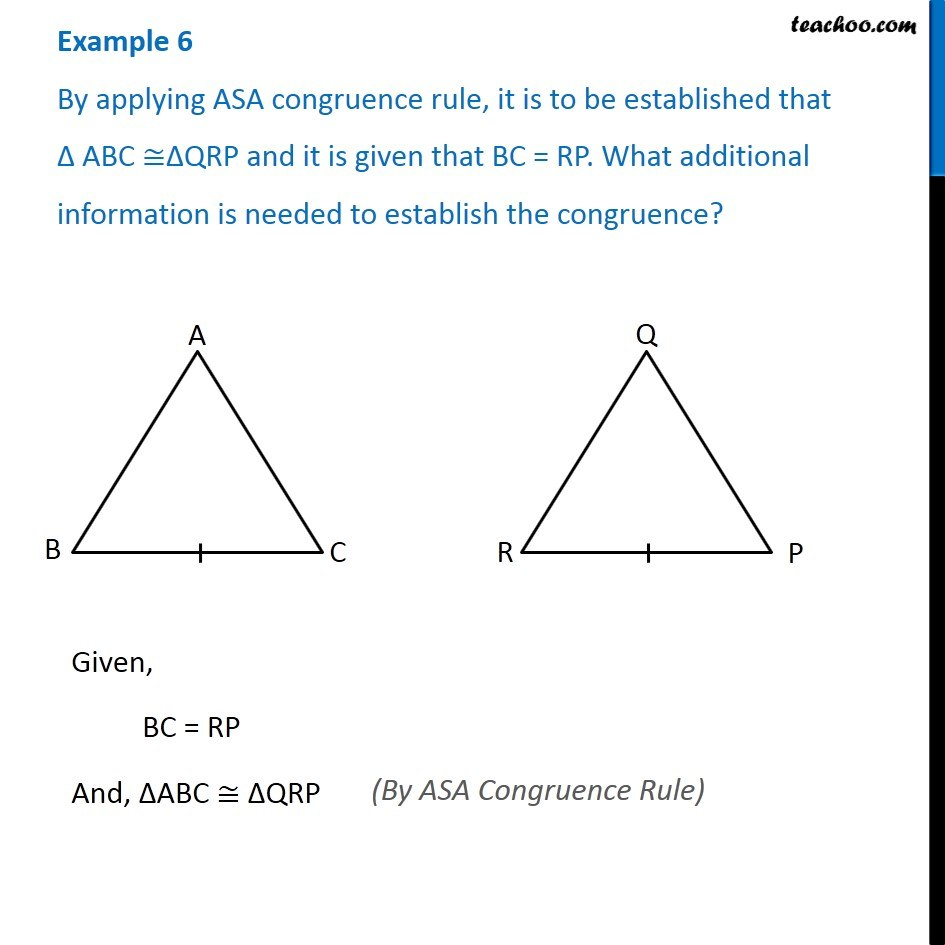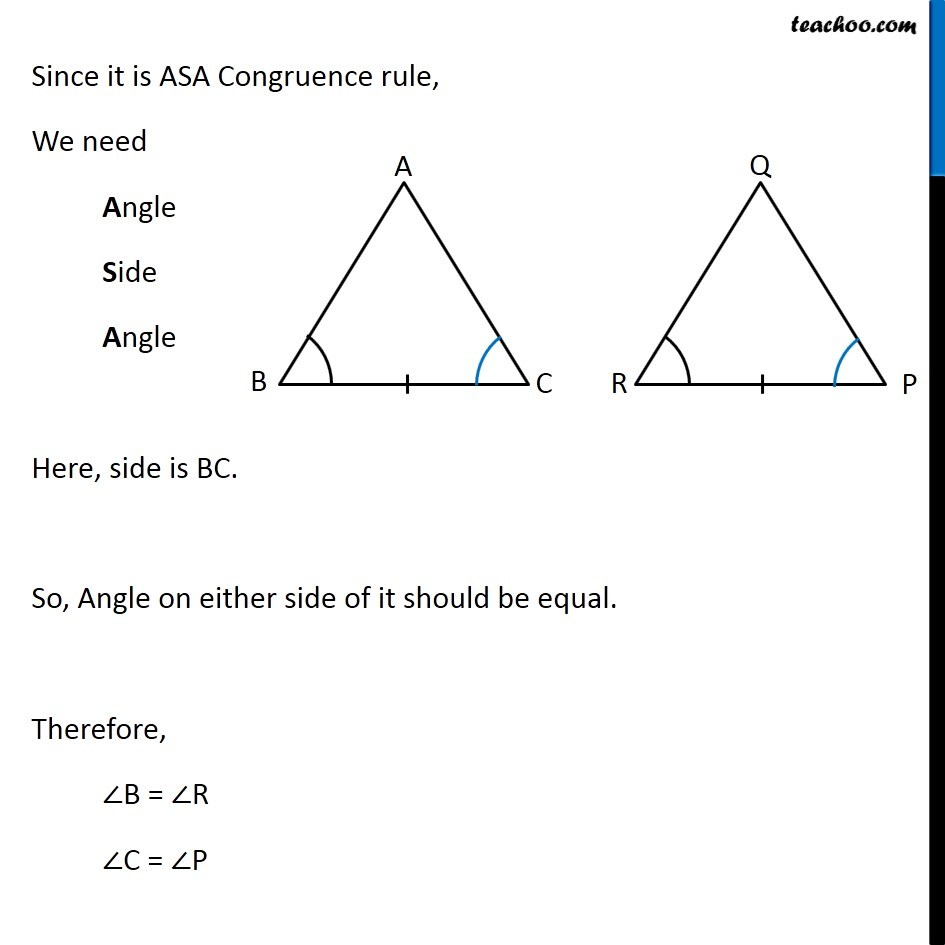Examples

Congruence of Triangles
Serial order wiseLearn in your speed, with individual attention - Teachoo Maths 1-on-1 Class

### Transcript

Example 6 - Chapter 7 Class 7 Congruence of Triangle - NCERT By applying ASA congruence rule, it is to be established that ∆ ABC ≅∆QRP and it is given that BC = RP. What additional information is needed to establish the congruence? Given, BC = RP And, ∆ABC ≅ ∆QRP (By ASA Congruence Rule) Since it is ASA Congruence rule, We need Angle Side Angle Here, side is BC. So, Angle on either side of it should be equal. Therefore, ∠B = ∠R ∠C = ∠P